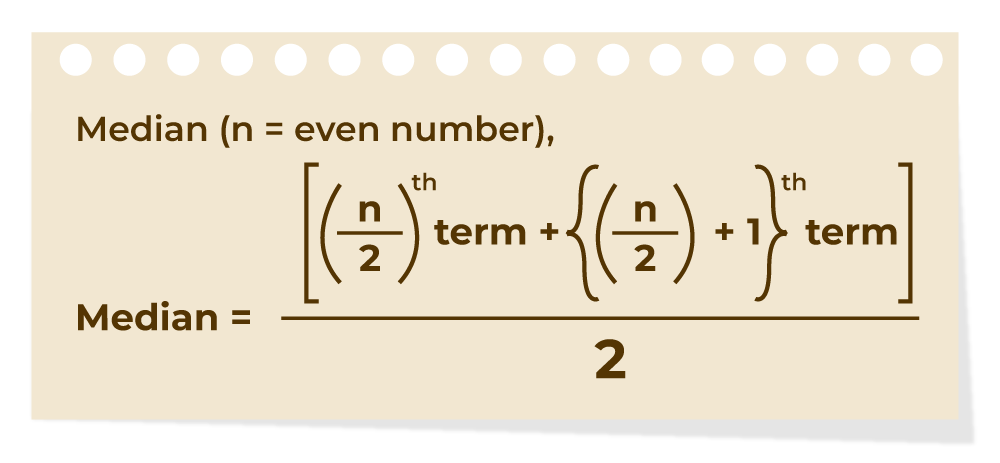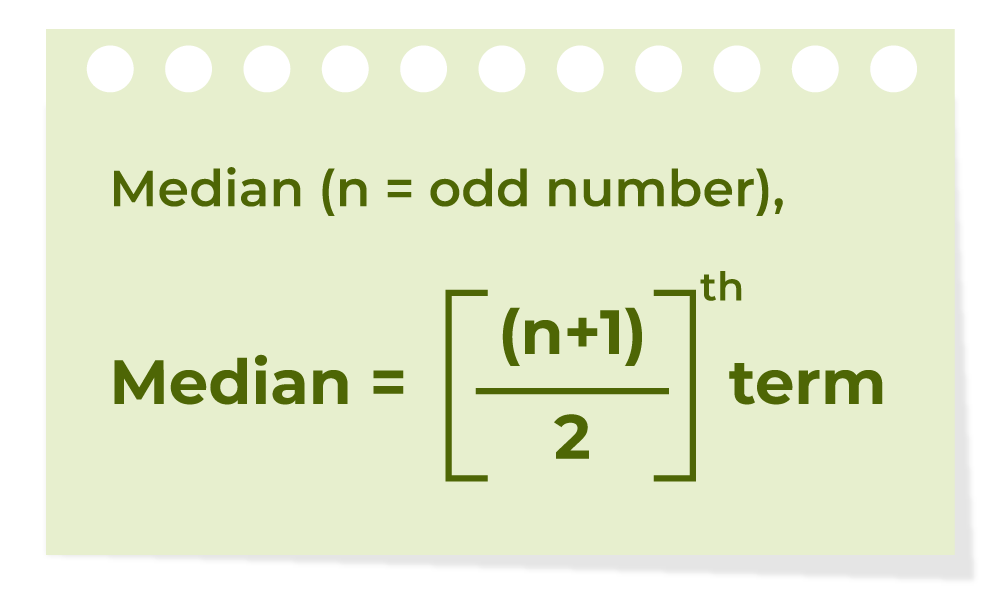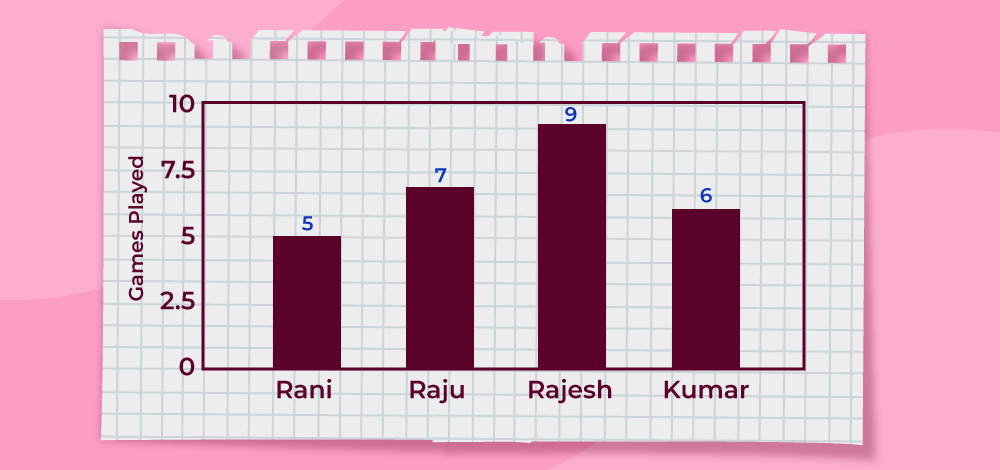Open In App
Related Articles
• CBSE Class 9 Maths Revision Notes
• CBSE Class 9 Maths Formulas
• NCERT Solutions for Class 9 Maths
• RD Sharma Class 9 Solutions

# Mean, Median and Mode

Mean, Median, and Mode are measures of the central tendency. These values are used to define the various parameters of the given data set. The measure of central tendency (Mean, Median, and Mode) gives useful insights about the data studied, these are used to study any type of data such as the average salary of employees in an organization, the median age of any class, the number of people who plays cricket in a sports club, etc.

## Measures of Central Tendency

Measure of central tendency is the representation of various values of the given data set. There are various measures of central tendency and the most important three measures of central tendency are,

• Mean (x̅ or μ)
• Median(M)
• Mode(Z)

## What is Mean?

Mean is the sum of all the values in the data set divided by the number of values in the data set. It is also called the Arithmetic Average. Mean is denoted as x̅ and is read as x bar.

The formula to calculate the mean is,### Mean Formula

The formula to calculate the mean is,

Mean (x̅)  = Sum of Values / Number of Values

If x1, x2, x3,……, xn are the values of a data set then the mean is calculated as:

x̅ =  (x1 + x2 + x3 + …… + xn) / n

Example: Find the mean of data sets 10, 30, 40, 20, and 50

Solution:

Mean of the data 10, 30, 40, 20, 50 is

Mean = (sum of all values) / (number of values)

Mean = (10 + 30 + 40 + 20+ 50) / 5

= 30

### Mean of Grouped Data

Mean for the grouped data can be calculated by using various methods. The most common methods used are discussed in the table below,

Direct MethodAssumed Mean MethodStep Deviation Method

Mean

x̅ = ∑ fixi / ∑ fi

where,
∑fi is the sum of all frequencies

Mean

x̅ = a + ∑ fixi / ∑ fi

where,
a is Assumed mean
di is equal to xi – a
∑fi the sum of all frequencies

Mean

x̅ = a + h∑ fixi / ∑ fi

where,
a is Assumed mean
ui = (xi – a)/h
h is Class size
∑fi the sum of all frequencies

Also, Check Mean

## What is Median?

A Median is a middle value for sorted data. The sorting of the data can be done either in ascending order or descending order. A median divides the data into two equal halves.

The formula to calculate the median of the number of terms if the number of terms is even is shown in the image below,The formula to calculate the median of the number of terms if the number of terms is odd is shown in the image below,### Median Formula

The formula for the median is,

If the number of values (n value) in the data set is odd then the formula to calculate the median is,

Median = [(n + 1)/2]th term

If the number of values (n value) in the data set is even then the formula to calculate the median is:

Median  = [(n/2)th term + {(n/2) + 1}th term] / 2

Example: Find the median of given data set 30, 40, 10, 20, and 50

Solution:

Median of the data 30, 40, 10, 20, 50 is,

Step 1: Order the given data in ascending order as:

10, 20, 30, 40, 50

Step 2: Check n (number of terms of data set) is even or odd and find the median of the data with respective ‘n’ value.

Step 3: Here, n = 5 (odd)

Median = [(n + 1)/2]th term

Median = [(5 + 1)/2]th term

= 30

### Median of Grouped Data

The median of the grouped data median is calculated using the formula,

Median = l + [(n/2 – cf) / f]×h

where
l is lower limit of median class
n is number of observations
f is frequency of median class
h is class size
cf is cumulative frequency of class preceding the median class.

## What is Mode?

A mode is the most frequent value or item of the data set. A data set can generally have one or more than one mode value. If the data set has one mode then it is called “Uni-modal”. Similarly, If the data set contains 2 modes then it is called “Bimodal” and if the data set contains 3 modes then it is known as “Trimodal”. If the data set consists of more than one mode then it is known as “multi-modal”(can be bimodal or trimodal). There is no mode for a data set if every number appears only once.

The formula to calculate the mode is shown in the image below,### Mode Formula

Mode = Highest Frequency Term

Example: Find the mode of the given data set 1, 2, 2, 2, 3, 3, 4, 5

Solution:

Given set is {1, 2, 2, 2, 3, 3, 4, 5}

As the above data set is arranged in ascending order.

By observing the above data set we can say that,

Mode = 2

As, it has highest frequency (3)

### Mode of Grouped Data

The mode of the grouped data is calculated using the formula,

Mode = l + [(f1 + f0) / (2f1 – f0 – f2)] × h

where,
f1 is the frequency of the modal class
f0 is the frequency of the class preceding the modal class
f2 is the frequency of the class succeeding the modal class
h is the size of class intervals
l is the lower limit of modal class

Also, Check Mode

## Relation between Mean Median Mode

For any group of data, the relation between the three central tendencies mean, median, and mode is shown in the image below,### Mean Median Mode Formula

Mode = 3 Median – 2 Mean

## Range

It is the difference between the highest value and the lowest value. It is a way to understand how the numbers are spread in a data set. The range of any data set is easily calculated by using the formula given in the image below,### Range Formula

The formula to find the Range is:

Range = Highest value – Lowest Value

Example: Find the range of the given data set 12, 19, 6, 2, 15, 4

Solution:

Given set is {12, 19, 6, 2, 15, 4}

Here,

Lowest Value = 2
Highest Value = 19

Range = 19 − 2
= 17

## Difference Between Mean and Median

The mathematical average is known as the mean of the data set, whereas the positional average is considered the Median.

The difference between Mean and Median is understood by the following example. In a school, there are 8 teachers whose salaries are 20000 rupees, a principal with a salary of 35000, find their mean salary and median salary.

Mean = (20000+20000+20000+20000+20000+20000+20000+20000+35000)/9
= 195000/9
= 21666.67

Therefore, the mean salary is ₹21,666.67.

For median, in ascending order: 20000, 20000, 20000, 20000, 20000, 20000, 20000, 20000, 35000.

n = 9,

Thus, (9 + 1)/2 = 5

Thus, the median is the 5th observation.

Median = 20000

Therefore, the median is ₹20,000.

After comparing the mean and median for the above data set. It is evident that the mean salary is Rs 21666.67, and the median is Rs 20,000

Note: Mean gets easily affected by extreme values.

Also, Check

## Examples on Mean, Median, and Mode

Example 1: Study the bar graph given below and find the mean, median, and mode of the given data set.Solution:

Mean = (sum of all data values) / (number of values)

Mean = (5 + 7 + 9 + 6) / 4
=  27 / 2
= 6.75

Order the given data in ascending order as: 5, 6, 7, 9

Here, n = 4 (which is even)

Median  =  [(n/2)th term + {(n/2) + 1}th term] / 2

Median  = (6 + 7) / 2
=  6.5

Mode = Most frequent value
= 9  (highest value)

Range = Highest value – Lowest value

Range = 9 – 5
= 4

Example 2: Find the mean, median, mode, and range for the given data

190, 153, 168, 179, 194, 153, 165, 187, 190, 170, 165, 189, 185, 153, 147, 161, 127, 180

Solution:

For Mean:

190, 153, 168, 179, 194, 153, 165, 187, 190, 170, 165, 189, 185, 153, 147, 161, 127, 180

Number of observations = 18

Mean = (Sum of observations) / (Number of observations)

= (190+153+168+179+194+153+165+187+190+170+165+189+185+153+147 +161+127+180) / 18

= 2871/18

= 159.5

Therefore, the mean is 159.5

For Median:

The ascending order of given observations is,

127, 147, 153, 153, 153, 161, 165, 165, 168, 170, 179, 180, 185, 187, 189, 190, 190, 194

Here, n = 18

Median = 1/2 [(n/2) + (n/2 + 1)]th observation
= 1/2 [9 + 10]th observation
= 1/2 (168 + 170)
= 338/2
= 169

Thus, the median is 169

For Mode:

The number with the highest frequency = 153

Thus, mode = 53

For Range:

Range = Highest value – Lowest value
= 194 – 127
= 67

Example 3: Find the Median of the data 25, 12, 5, 24, 15, 22, 23, 25

Solution:

25, 12, 5, 24, 15, 22, 23, 25

Step 1: Order the given data in ascending order as:

5, 12, 15, 22, 23, 24, 25, 25

Step 2: Check n (number of terms of data set) is even or odd and find the median of the data with respective ‘n’ value.

Step 3: Here, n = 8 (even) then,

Median = [(n/2)th term + {(n/2) + 1)th term] / 2

Median = [(8/2)th term + {(8/2) + 1}th term] / 2

= (22+23) / 2

= 22.5

Example 4: Find the mode of given data 15, 42, 65, 65, 95.

Solution:

Given data set 15, 42, 65, 65, 95

The number with highest frequency = 65

Mode = 65

## FAQs on Mean, Median, and Mode

### Q1: What are the mean, median, and mode?

Mean, Median and Mode are the measures of central tendency. These three measures of central tendency are used to get an overview of the data. They represent the true essence of the given data set.

### Q2: What is the relation between mean, median, and mode?

The relationship between mean median and mode is:

Mode = 3 Median – 2 Mean

### Q3: How to Find Mean, Median, and Mode?

Mean, Median, and Mode of any given data set is calculated using the suitable formulas which are discussed above in the articles.

### Q4: How to Find the mean?

Mean is also called the average, it is calculated for ungrouped data using the formula:

• Mean = (Sum of observations)/(Number of observations)

In case of Grouped Data, the mean is calculated by the three methods

• Direct method
• Assumed mean method
• Step deviation method